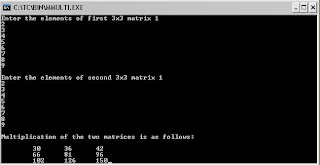## Friday, December 10, 2010

### C program for matrix multiplication of two matrix

Hello everybody I want to discuss the source code for matrix multiplication in c programming.## Program Code

`//C program for multiplication of two matrix#include < stdio.h >#include < conio.h >void main(){ int a, b, c, i, j, k; clrscr(); printf("Enter the elements of first 3x3 matrix"); for (i = 0;i < 3; i++) {  for (j = 0; j < 3; j++)  {   scanf("%d",&a[i][j]);  } } printf("\nEnter the elements of second 3x3 matrix"); for(i = 0; i < 3; i++) {  for (j = 0; j < 3; j++)  {   scanf("%d", &b[i][j]);  } } printf("\nThe first matrix is :-\n"); for (i = 0; i < 3; i++) {  for (j = 0; j < 3; j++)  {   printf("\t%d", a[i][j]);  }  printf("\n"); } printf("\nThe second matrix is :-\n"); for (i = 0; i < 3; i++) {  for (j = 0; j < 3; j++)  {   printf("\t%d", b[i][j]);  }  printf("\n"); } printf("\nMultiplication of the two matrices is as follows:\n"); for (i = 0;i < 3; i++) {  printf("\n");  for (j = 0; j < 3; j++)  {   c[i][j]=0;   for(k=0;k<3;k++)   c[i][j] = c[i][j]+a[i][k] * b[k][j];   printf("\t%d", c[i][j]);  } } getch();}`

## Input :

Enter the elements of first 3x3 matrix

1
2
3
4
5
6
7
8
9
Enter the elements of second 3x3 matrix
1
2
3
4
5
6
7
8
9

## Output :

Multiplication of the two matrices is as follows

30 36 42
66 81 96
102 126 150